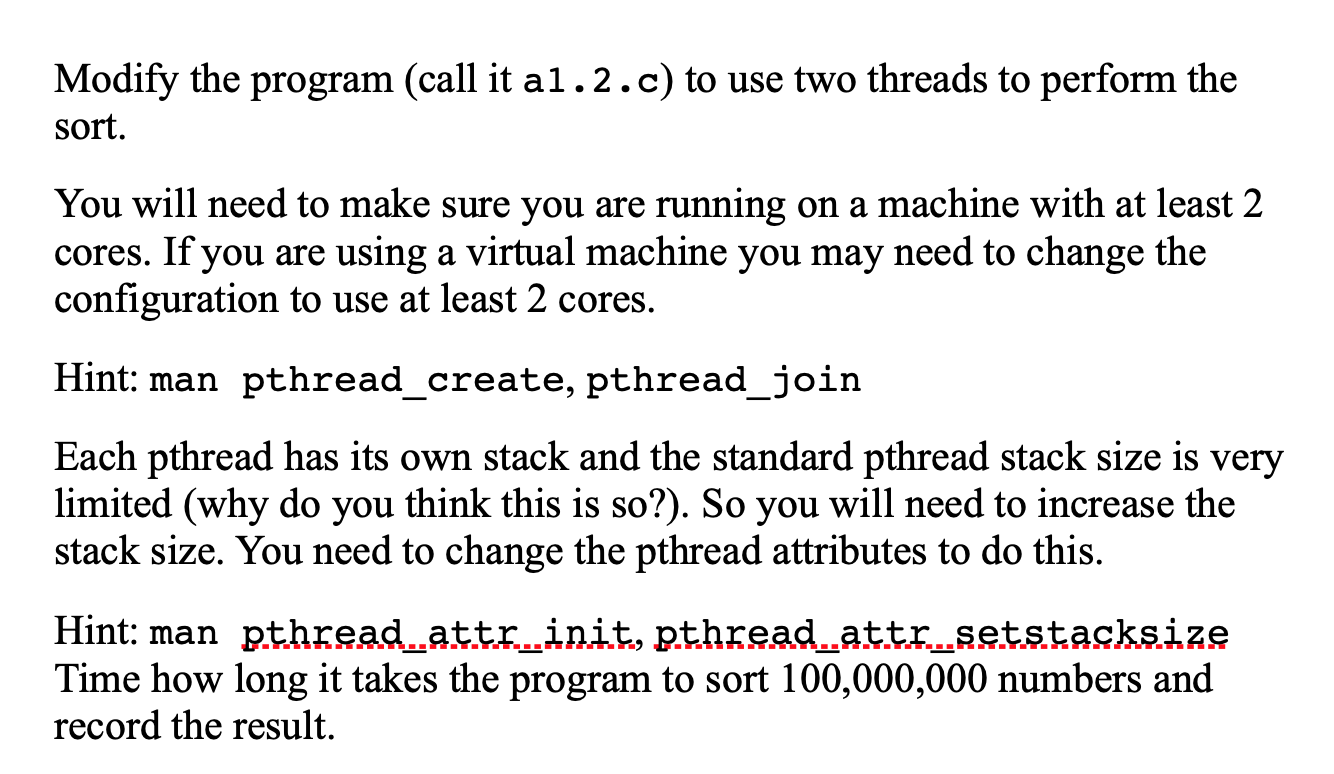### c programming question code to modify include include include include include includ 5150159C programming question:Code to modify:

#include
#include
#include
#include
#include
#include

#define SIZE 3

struct block {
int size;
int *first;
};

// void print_block_data(struct block *blk) {
// printf(“size: %d address: %pn”, blk->size, blk->first);
// }

/* Combine the two halves back together. */
void merge(struct block *left, struct block *right) {
int combined[left->size + right->size];
int dest = 0, l = 0, r = 0;
while (l < left->size && r < right->size) {
if (left->first[l] < right->first[r])
combined[dest++] = left->first[l++];
else
combined[dest++] = right->first[r++];
}
while (l < left->size)
combined[dest++] = left->first[l++];
while (r < right->size)
combined[dest++] = right->first[r++];
memmove(left->first, combined, (left->size + right->size) * sizeof(int));
}

/* Merge sort the data. */
void merge_sort(struct block *my_data) {
// print_block_data(my_data);
if (my_data->size > 1) {
struct block left_block;
struct block right_block;
left_block.size = my_data->size / 2;
left_block.first = my_data->first;
right_block.size = left_block.size + (my_data->size % 2);
right_block.first = my_data->first + left_block.size;
merge_sort(&left_block);
merge_sort(&right_block);
merge(&left_block, &right_block);
}
}

/* Check to see if the data is sorted. */
bool is_sorted(int data[], int size) {
bool sorted = true;
for (int i = 0; i
if (data[i] > data[i + 1])
sorted = false;
}
return sorted;
}

int main(int argc, char *argv[]) {
long size;

if (argc
size = SIZE;
} else {
size = atol(argv);
}
struct block start_block;
int data[size];
start_block.size = size;
start_block.first = data;
for (int i = 0; i
data[i] = rand();
}
printf(“starting—n”);
merge_sort(&start_block);
printf(“—endingn”);
printf(is_sorted(data, size) ? “sortedn” : “not sortedn”);
exit(EXIT_SUCCESS);
}

Modify the program (call it al.2.c) to use two threads to perform the sort. You will need to make sure you are running on a machine with at least 2 cores. If you are using a virtual machine you may need to change the configuration to use at least 2 cores. Hint: man pthread_create, pthread_join Each pthread has its own stack and the standard pthread stack size is very limited (why do you think this is so?). So you will need to increase the stack size. You need to change the pthread attributes to do this. Hint: man pthread_attr_init, pthread_attr_setstacksize Time how long it takes the program to sort 100,000,000 numbers and record the result.